### Home > PC > Chapter 2 > Lesson 2.3.7 > Problem2-157

2-157.
1.Jill has a job like Jack’s except that she makes $10/hr after six hours. Homework Help ✎ 1. Find J(t), Jill’s earnings after t hours of work. 2. Crown’s job pays$6/hr for the first 8 hours and then $12/hr after that. What is C(t), Crown’s earnings after t hours on the job? 3. When will Jill make$46?

4. Extension: When does Jill make more than Crown?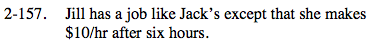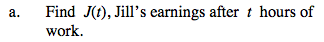$$J(t) = \left\{ \begin{array}{l l} 5t & \quad \text{for }0\le t \le 6\\ 30+10(\textit{t}-6) & \quad \text{for\,\textit{t}>6} \end{array} \right.$$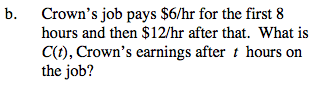Use the same thinking as in (a) above.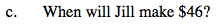You know that in 6 hours
Jill will have \$30.
So 30 +10(t − 6) = 46.
Solve for t.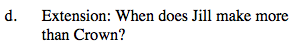When is 30 + 10(t − 6) > 48 + 12(t − 8) AND 6t < 30 + 10(t − 6)?